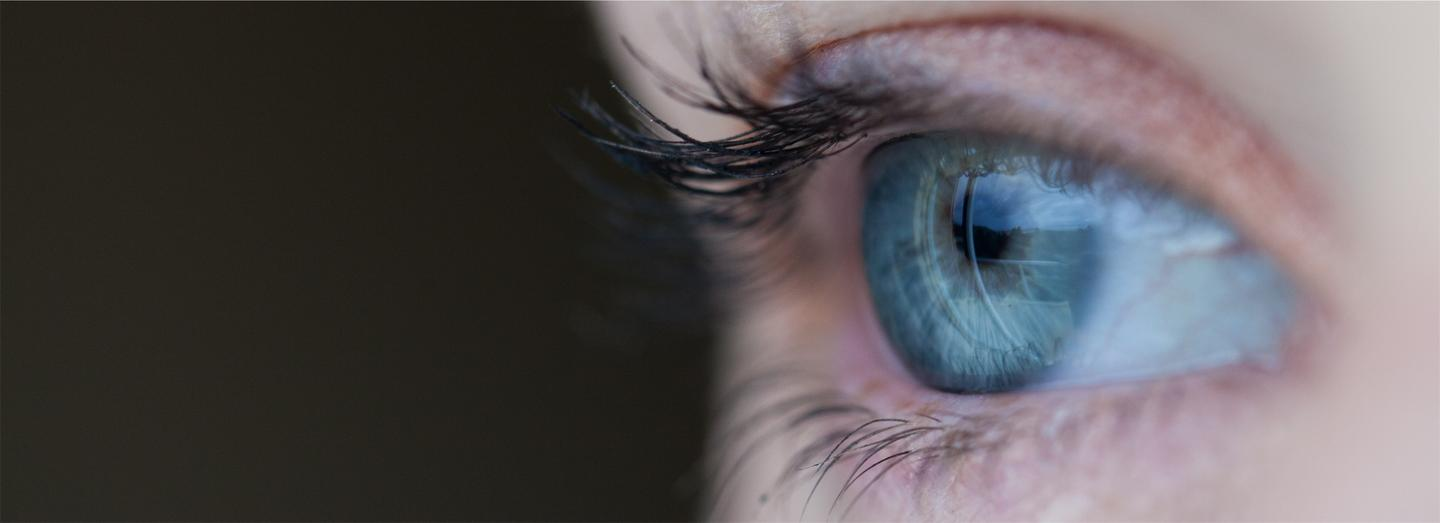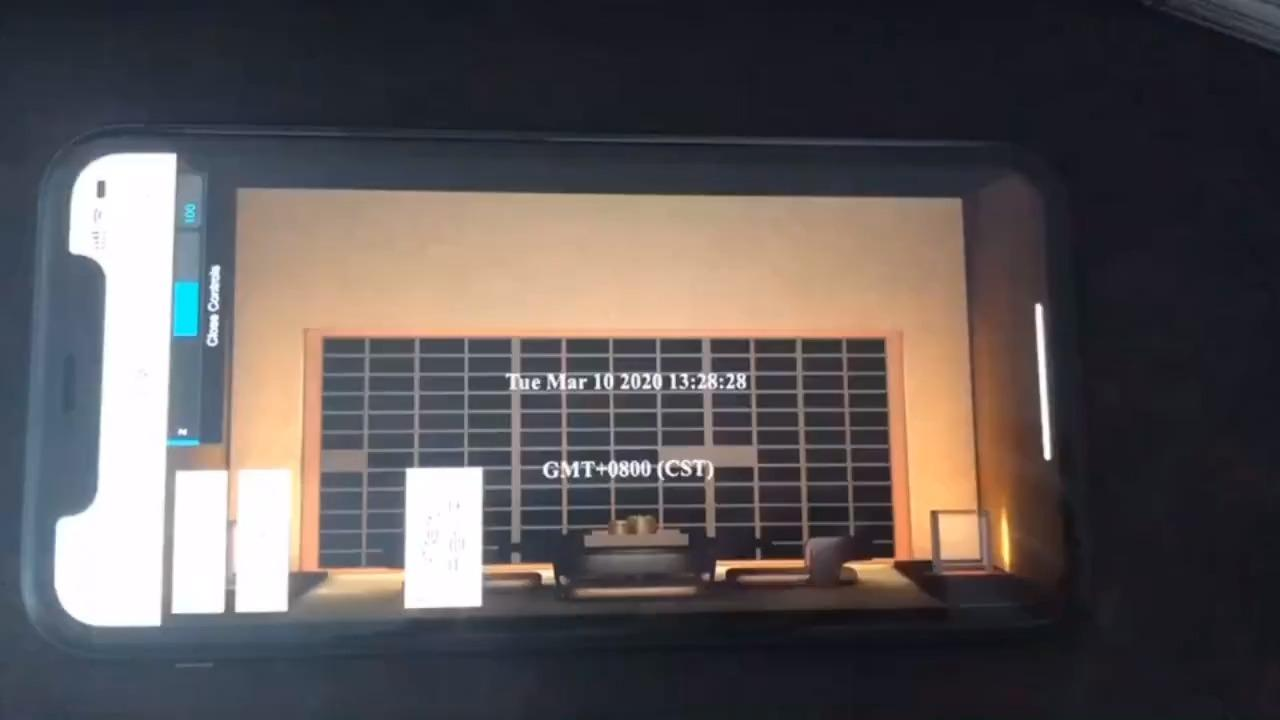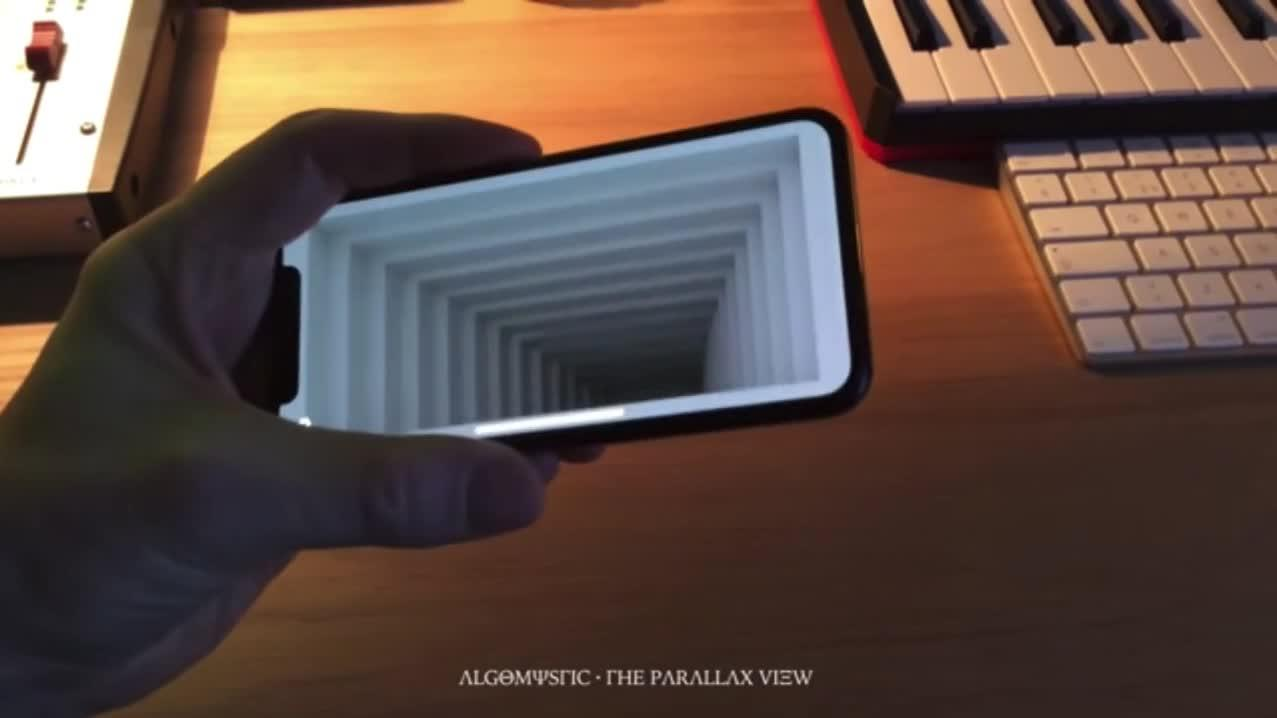https://www.zhihu.com/video/1225462082201702400

``````<script
src="https://game.gtimg.cn/images/js/sign/glassfree3d/js/GlassFree3dCamera.js"
></script>
new THREE.GlassFree3dCamera(x, y, z, width, height);
``````

## 实现原理https://www.zhihu.com/video/1225462220651495424

“盒子”的四个角始终“”在画面的四个角上。

## 3d相机机制

3d相机的算法核心是投影矩阵：

three.js的投影矩阵：

``````var te = [];
var x = 2 \* near / ( right - left );
var y = 2 \* near / ( top - bottom );

var a = ( right + left ) / ( right - left );
var b = ( top + bottom ) / ( top - bottom );
var c = - ( far + near ) / ( far - near );
var d = - 2 \* far \* near / ( far - near );

te[ 0 ] = x; te[ 4 ] = 0; te[ 8 ] = a; te[ 12 ] = 0;
te[ 1 ] = 0; te[ 5 ] = y; te[ 9 ] = b; te[ 13 ] = 0;
te[ 2 ] = 0; te[ 6 ] = 0; te[ 10 ] = c; te[ 14 ] = d;
te[ 3 ] = 0; te[ 7 ] = 0; te[ 11 ] = - 1; te[ 15 ] = 0;
``````

glassfree3dcamera组件中，添加了touch事件进行绑定，通过下面语句开启点击事件。

``````camera.initMove();
``````

``````camera.initDeviceOrientation();
``````

## 手机的朝向与陀螺仪

gamma角是手机的x轴与地面的角度值

alpha与手机的轴无关，beta，gamma值与手机当前yx轴的位置相关，而另外一个很容易让人误解的就是谷歌开发者工具里的sensors：

``````var quaternion = new THREE.Quaternion();
var euler= new THREE.Euler();
euler.set( beta, alpha, - gamma, 'YXZ' );
quaternion.setFromEuler( euler );
``````

### 结语：

anxious-bored.com/blog/

songho.ca/opengl/gl_pro

bilibili.com/video/av90

eater.net/quaternions